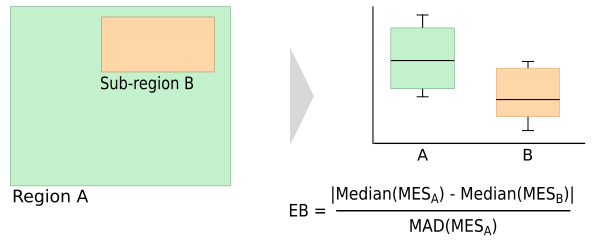## NAME

r.meb - Compute the multivariate environmental bias (MEB)

## KEYWORDS

similarity, raster, modelling

## SYNOPSIS

r.meb
r.meb --help
r.meb [-imno] env=names[,names,...] ref=names [output=names] [file=name] [digits=string] [--overwrite] [--help] [--verbose] [--quiet] [--ui]

### Flags:

-i
Compute EB for individual variables
-m
Use mean values of IES layers to compute MES
-n
Use median values of IES layers to compute MES
-o
Use minimum values of IES layers to compute MES
--overwrite
Allow output files to overwrite existing files
--help
Print usage summary
--verbose
Verbose module output
--quiet
Quiet module output
--ui
Force launching GUI dialog

### Parameters:

env=names[,names,...] [required]
Environmental layers
Raster map(s) of environmental conditions
ref=names [required]
Reference area
Sub-area (1) within region (1+0) for which to compute the EB
output=names
Root of name output layers
Output MES layer (and root for IES layers if kept)
file=name
Name of output text file
Name of output text file (csv format)
digits=string
Precision of your input layers values
Default: 5

## DESCRIPTION

The Multivariate Environmental Bias (MEB) takes the medium conditions in an area N and computes how much conditions in a subset of N (S) deviate from these medium conditions (van Breugel et al. 2015). This can for example be used to see how well conditions in the protected areas of a country represent conditions in the whole country.

The messure is based on the Multivariate Environmental Similarity (MES) surface, which was proposed by Elith et al (2015). To compute the MES first the similarity of a point P to the conditions in N with respect to variable V is computed. The similarity is expressed as the deviation from the median of V in P to those in N. This is done for all variables of interest (V1, V2, ...Vj).

In the original equation proposed by Elith et al (2010) the final MES of P is computed as the minimum of the similarity values (IESminimum) of the individual variables (Vj) in P. However, to compute the MEB using the mean or median may be a better choice as they take into account the similarity along all environmnetal axes and not only the one that deviates most. The user therefore has the option to use the mean (IESmean) or median (IESmedian) of the IES instead (there is still the option to use the minimum as well).

The MEB is computed as the absolute difference of the median of the MES in the whole target area (MESn) and the median of the MES in the subset (MESs), divided by the median absolute deviation (MAD ) of the MES in N. It is also possible to compute the bias based on the individual variables (IEB) in which case the IES instead of the MES is used.The addon creates a MES layer and a table (saved to csv file) with the median value of each variable in the region and in the reference area, the median absolute deviation (mad) and the environmental bias (eb). Optionally, this can be computed for the individual variables as well. The user has the option to have the addon compute the MEB based on the MES computed using the minimum, average and/or median of the IES layers (see above)

## NOTE

Input variables are expected to be or to represent continuous variables.

## CITATION

van Breugel P, Kindt R, Lillesø J-PB, van Breugel M (2015) Environmental Gap Analysis to Prioritize Conservation Efforts in Eastern Africa. PLoS ONE 10(4): e0121444. doi: 10.1371/journal.pone.0121444

## Example

In the example below the MEB for a map 'forestmap' which gives the distribution of forest (1) and other land cover types (0). As environmental variables, the bio1, bio3 and bio9 are used.
```r.meb -m -n -o env=bio_1,bio_3,bio_9 ref=forestmap output=Test file=Test
Median Test_MES_mean (all region) = 47.338
Median Test_MES_mean (ref. area) = 69.798
MAD Test_MES_mean (all region) = 14.594
EB = 1.539

Median Test_MES_median (all region) = 45.712
Median Test_MES_median (ref. area) = 69.897
MAD Test_MES_median (all region) = 18.786
EB = 1.287

Median Test_MES_minimum (all region) = 20.364
Median Test_MES_minimum (ref. area) = 55.807
MAD Test_MES_minimum (all region) = 15.096
EB = 2.348

The results are written to Test.csv
```

## CITATION

Lillesø J-PB, van Breugel M (2015) Environmental Gap Analysis to Prioritize Conservation Efforts in Eastern Africa. PLoS ONE 10(4): e0121444. doi: 10.1371/journal.pone.0121444

## REFERENCES

• Elith, J, Kearney, M, and Phillips, S. 2010. The art of modelling range-shifting species. Methods in Ecology and Evolution 1:330-342.
• van Breugel P, Kindt R, Lillesø J-PB, van Breugel M. 2015. Environmental Gap Analysis to Prioritize Conservation Efforts in Eastern Africa. PLoS ONE 10(4): e0121444. doi: 10.1371/journal.pone.0121444.